+

# Slope in Context

Author: Sophia Tutorial
##### Description:

Calculate the average rate of change in a given scenario.

(more)

Sophia’s self-paced online courses are a great way to save time and money as you earn credits eligible for transfer to many different colleges and universities.*

No credit card required

31 Sophia partners guarantee credit transfer.

302 Institutions have accepted or given pre-approval for credit transfer.

* The American Council on Education's College Credit Recommendation Service (ACE Credit®) has evaluated and recommended college credit for 30 of Sophia’s online courses. Many different colleges and universities consider ACE CREDIT recommendations in determining the applicability to their course and degree programs.

Tutorial
what's covered
1. Average Rate of Change
2. Average Rate of Change and Slope
3. Positive, Negative, and Zero Slope

# 1. Average Rate of Change

Imagine that we are planning a trip from Chicago, IL to Knoxville, TN. The most direct flight covers a distance of 475 miles in 1 hour. Dividing distance by time, we get a speed of 475 miles per hour. This represents the average speed, or average rate of change in distance over change in time. In reality, the plane travels at varying speeds, particularly during take off and landing, but generally speaking, we say that the airplane travels at a speed of 475 miles per hour.

On a graph, we see that the average speed is represented by a straight line, while the actually path of the plane may be non-linear: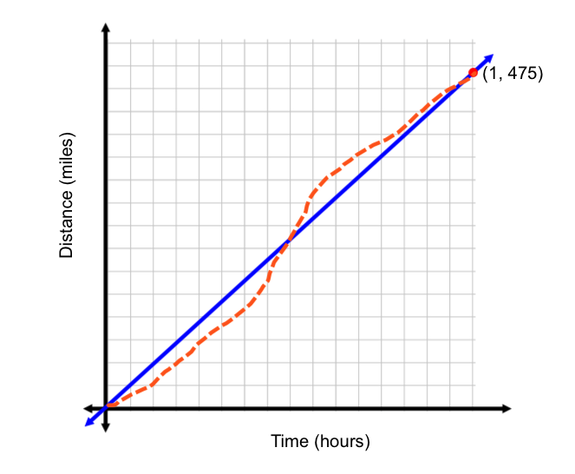# 2. Average Rate of Change and Slope

As we can see from the graph above, the average speed of the airplane is the slope of the line. Recall that the algebraic definition of slope is change in y-values divided by the change in x-values. In this scenario, the change in y-values is 475, because the starting distance was 0 miles and the ending distance was 475 miles. Our change in x-values is 1, because at 0 hours we were at 0 miles traveled, and at 1 hour we were at 475 miles traveled.

big idea
Average Rate of Change = Slope

EXAMPLE

Kristen is hiking on a local trail. While hiking, she decides to use her GPS to track her elevation. When she reaches mile marker 2, she measured her elevation at 4,100 feet. Once she reached mile marker 5, her elevation was now at 5,300 feet. Let's find the average change in elevation of Kristin's position from mile marker 2 to mile marker 12.
To find average change, we need to figure out what the elevation over the mile marker distance would be. When considering her elevation, we will look at the change between each mile marker. From mile marker 2 to mile marker 12, Kristen went from 4,100 feet to 5,300 feet. This is a difference of (5,300 - 4,100) = 1,200 feet in elevation.
The difference in mile marker distance was from mile marker 2 to mile marker 5, or a difference of (5 - 2) = 3.
We know that Kristen's elevation changed 1,200 feet in 3 mile markers. To simplify this, we just need to divide change in elevation by the change in mile marker.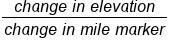Average rate of change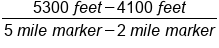Plug in elevation at mile marker 2 and 5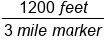Subtract the numerator and denominator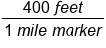Divide numerator by denominator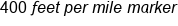Our solution

We know that Kristin traveled a total of 1,200 feet in elevation. In that same amount of time on her hike, she traveled a total of 3 miles. To simplifying this, we know that 1,200 divided by 3 is going to give us 400. The units for average change will be 400 feet for every 1 mile marker.

# 3. Positive, Negative, and Zero Slope

Take a look at the graphs below: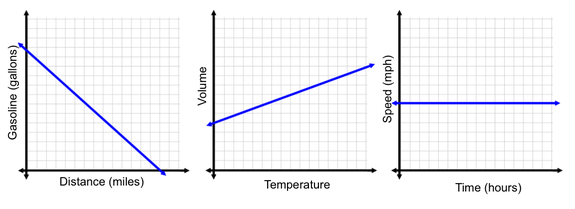In the graph above, we have one graph with a negative slope, a graph with a positive slope, and a graph with a slope of zero. Let's interpret the slope within the context that's provided by the information in the axes.

Negative Slope

The first graph shows a relationship between distance traveled and gallons of gasoline that a car has in its tank. The negative slope indicates that as the car continues to travel, fewer and fewer gallons of gasoline remain in the tank. We can interpret the y-intercept as the amount of gasoline the car started with, and the x-axis as the number of miles the car travels until it runs out of gas.

Positive Slope

The graph in the middle shows a relationship between the volume of an object and the temperature of its environment. The positive slope indicates that as the room temperature increases, or as the object gets warmer, it expands, increasing in volume.

Zero Slope

The third graph shows a relationship between the amount of time an object travels and its speed. The slope of zero indicates that there is no change in speed during the time interval recorded on this graph. We could perhaps interpret this graph as being a car on the highway set in cruise control. When a car is set in cruise control, its speed is locked, and will continue to travel at a constant speed. With a constant speed, there is no change in speed from one time interval to the next.

summary
You can use the total time and total distance traveled to calculate an object's average speed. The average rate of change, or speed, describes a constant rate the object traveled to cover a distance over a certain period of time. When comparing the average rate of change and slope, the slope of a line that represents an object's type and distance will equal the average rate of change or speed of the object. In context, we can identify three types of slope: positive, negative, and zero slope. A negative slope would indicate as one variable increases, the other variable decreases. A positive slope would indicate as one variable increases, the other variable also increases. A zero slope indicates that there is no change in one variable as the other variable changes.

Rating